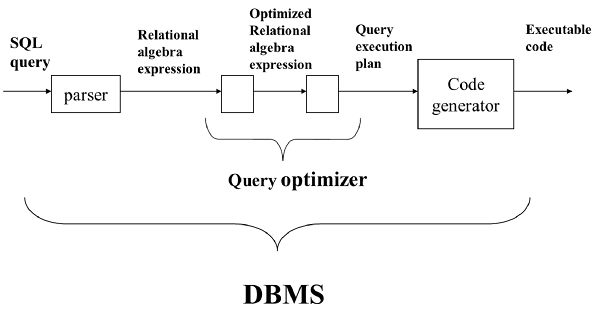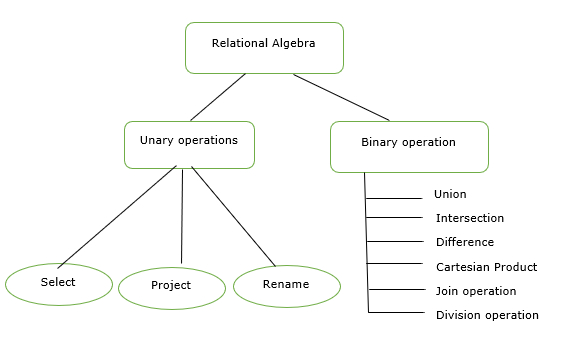# Explain the relational algebra in DBMS?

Query is a question or requesting information. Query language is a language which is used to retrieve information from a database.

## Relational algebra

It is a procedural query language. It gives a step-by-step process to obtain the result of the query. It uses operators to perform queries.

Relational algebra can be depicted as follows −## Algebraic Operations

The different algebraic operations in the DBMS are as follows −Let us understand the relational algebra operations in DBMS.

## Select operation

It displays the records that satisfy a condition. It is denoted by sigma (σ). It is a horizontal subset of the original relation.

### Syntax

σcondition(table name)

## Projection operation

It displays the specific column of a table. It is denoted by pie (П). It is a vertical subset of the original relation. It eliminates duplicate tuples.

### Syntax

Пregno(student)

## Rename operation

It is used to assign a new name to a relation. It is denoted by ρ (rho).

### Syntax

ρnewname (tablename or expression)

## Union operation

Union operation combines values in R1, R2 removing duplicate ones.

### Syntax

Пregno(R1) ∪ Пregno(R2)

It displays all the regno of R1 and R2.

## Intersection operation

It displays the common values in R1 & R2 and is denoted by ∩.

### Syntax

Пregno(R1) ∩ Пregno(R2)

## Difference operation

It displays the value present in R1 but not in R2. It is denoted by –(minus).

### Syntax

Пregno(R1) - Пregno(R2)

### Cartesian product operation

It combines R1 and R2 without any condition and is denoted by X.

Degree of R1 XR2 = degree of R1 + degree of R2

{degree = total no of columns}

Join operation

It combines R1 and R2 with respect to a condition. It is denoted by ⋈.

Joins are of different types

• Theta join.

• Natural join.

• Outer join − This is further classified into the following types −

• Left outer join.

• Right outer join.

• Full outer join.

Division operation

The division operator is used for queries which involves the ‘all’

R1/R2 = tuples of R1 associated with all tuples of R2.